Mobile QR Code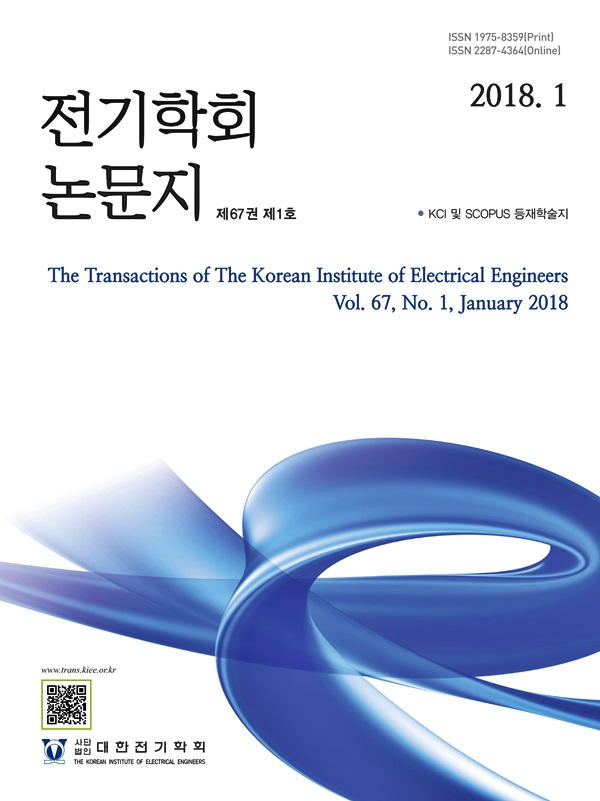#### The Transactions of the Korean Institute of Electrical Engineers

##### ISO Journal TitleTrans. Korean. Inst. Elect. Eng.
• SCOPUS
• KCI Accredited Journal

1. (Dept. of Electrical Engineering, Chosun University, Korea.)

Superconducting element, LC divergence oscillation, DC circuit breaker, PSCAD/EMTDC

## 1. 서 론

In order to reduce carbon emissions worldwide, the scope of use of new and renewable energy sources is expanded, and policies are being implemented to pursue changes in electric models in internal combustion engines. As the number and capacity of new and renewable energy sources are expanded, the range of DC systems is also expanding. Recently, a project is underway to expand the multiplexed structure from LVDC to HVDC systems. As such, the number and capacity of DC loads increase, and the appearance of the multiplexed structure of the DC system is likely to be the current state. Therefore, we have to prepare for the transient state of the DC grid in accordance with the multiplexed structure of the DC grid network (2).

We are conducting a research on a DC cut-off technology based on the most representative solar power of renewable energy for reliable system operation. The mechanical DC circuit breaker used has a structure suitable for the low breaking capacity of the LVDC. However, it is expected that several generation facilities of the solar power will gather and link to form a highly reliable system. Then, a multiplexed photovoltaic grid is formed, and when an unavoidable failure occurs for the DC system, a higher fault current is generated than that of a single system failure. We are working on to the solar power system to which the existing mechanical DC circuit breaker is connected. Also, we are working on to confirm whether the DC system can be a reliable DC system even with the change of the multiplex system by applying the LC divergence oscillation circuit and the superconducting element (3-5). We proposed a model that combines a superconducting current limiter, a mechani- cal DC circuit breaker, and an LC divergent vibration circuit. The superconducting element can reduce the high initial fault current within about 2 ms through the quench. Its material is GdBCO. Mechanical DC circuit breaker has no power loss and higher mechanical strength than semiconductor switch. Therefore, it is a switching structure that can strongly respond to the DC fault current. However, due to the relatively slow the opening speed of the cut-off contact, it cannot respond directly to the rapid growth of the DC fault current. To solve this problem, we used the superconducting element with a mechanical DC circuit breaker. We want to analyze the characteristics of the cut-off operation generated through the hybrid method and determine the suitability. We analyze the operation characteristics of the mechanical DC circuit breaker used in solar power system. Also, based on this data, we analyze the cut-off operation characteristics of the model applying the superconducting element and the LC divergent oscillation circuit are analyzed through PSCAD. In addition, a simulation analysis of the breaking capacity of the model applying the superconducting element and the LC divergent oscillation circuit will be conducted.

## 2. 본 론

### 2.1 Operating characteristics of the mechanical DC circuit breaker

An experiment was conducted on the operating characteristics of a mechanical DC circuit breaker applied to a solar power system. The mechanical DC circuit breaker model is BK63H DC, and it has a terminal structure of the lug type and a method of the thermal-magnetic overcurrent trip. A rated voltage is 500 V and a rated current is 50 A. The rated voltage and current were set and tested in the DC simulation system. We simulated a short circuit by quickly switching between a normal line and a transient line using the switch of the SCR semiconductor type. The normal load connected to the normal line is about 10 Ω and the fault load connected to the transient line is about 1 Ω. Figure 1 is a graph of the cut-off operation characteristics shown the experiment data of a mechanical DC cut-off switch through a short-circuit fault. As the test system, a rated voltage and current are about 500 V and 50 A was set. Figure 1-(a) is about 19.4 ms when the fault occurred. The initial fault current generated through the experiment was generated from about 47 A to about 501.6 A for about 0.3 ms. This is the rate of increase of the HVDC fault current and the time constant is about 0.1.

Figure 1-(b) shows the time when about 14.85 ms passes after the fault current occurs and the opening operation of the mechanical DC circuit breaker occurs. From this point on, an arc voltage is generated by the opening operation of the cut-off contact, and the fault current is reduced. Figure 1-(c) is point of the cut-off operation completed about 54.16 ms. When the fault current was completed, a transient recovery voltage occurred, which occurred from about 54.25 ms to about 639.5 V. This occurred at about 54.95 ms with a rated voltage of about 500.7 V. The total time of the shutdown was about 35.55 ms due to the single shutdown operation of the mechanical DC circuit breaker.

그림 1 초전도 소자와 LC발산진동회로가 없는 기계식 차단기의 동작 특성 실험 그래프

Fig. 1 Graph of experimental results of mechanical DC circuit breaker without the superconducting element and LC divergence oscillation circuit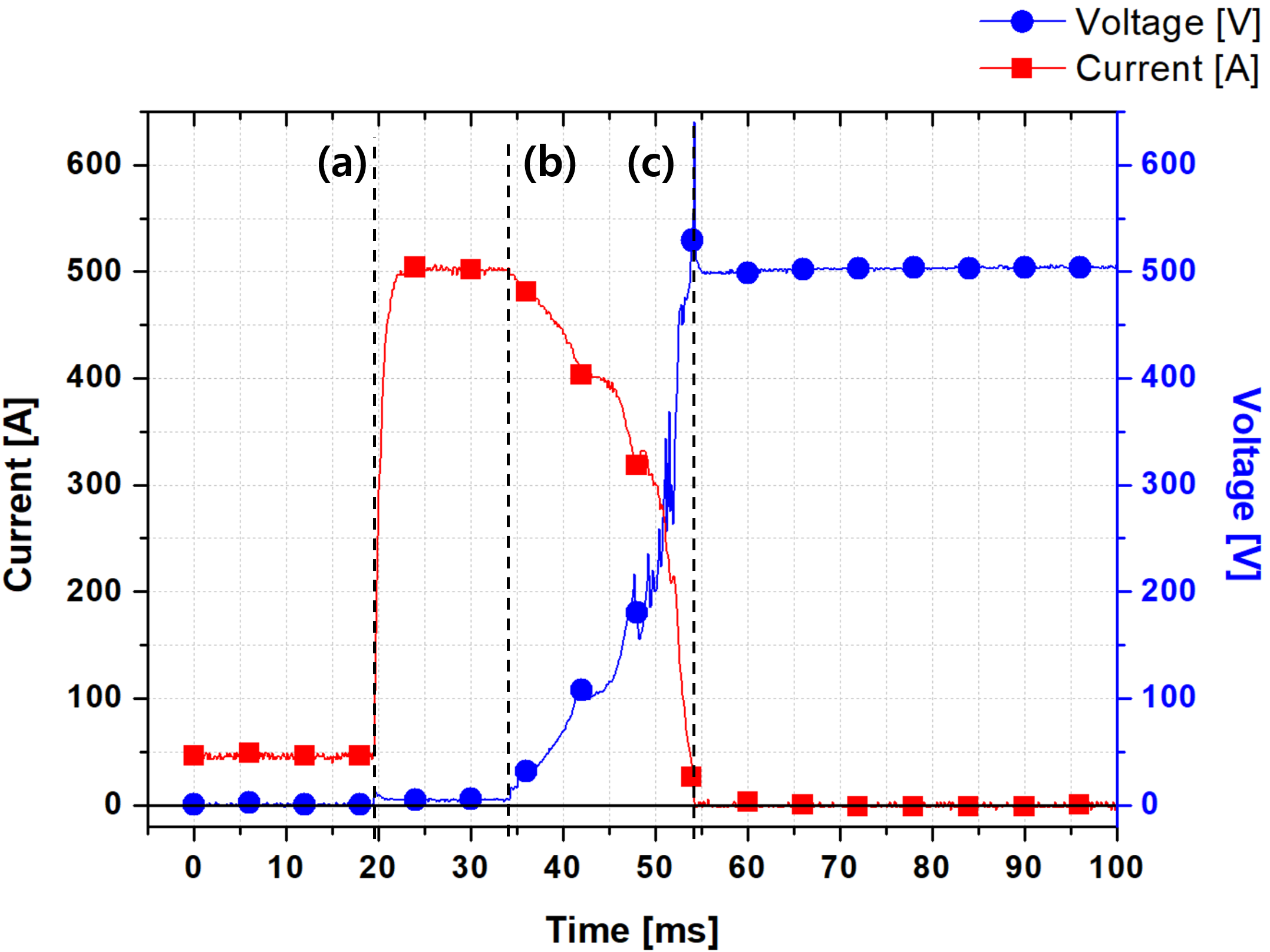### 2.2 The operating characteristics of a mechanical DC circuit breaker that combines the LC diver- gent oscillation circuit and SPD

그림 2 초전도 소자가 없는 기계식 LC 발산진동회로가 적용된 기계식 차단기 동작 특성 실험 결과 그래프

Fig. 2 Graph of experimental results of mechanical DC circuit breaker applied LC divergence oscillation circuit without the superconducting element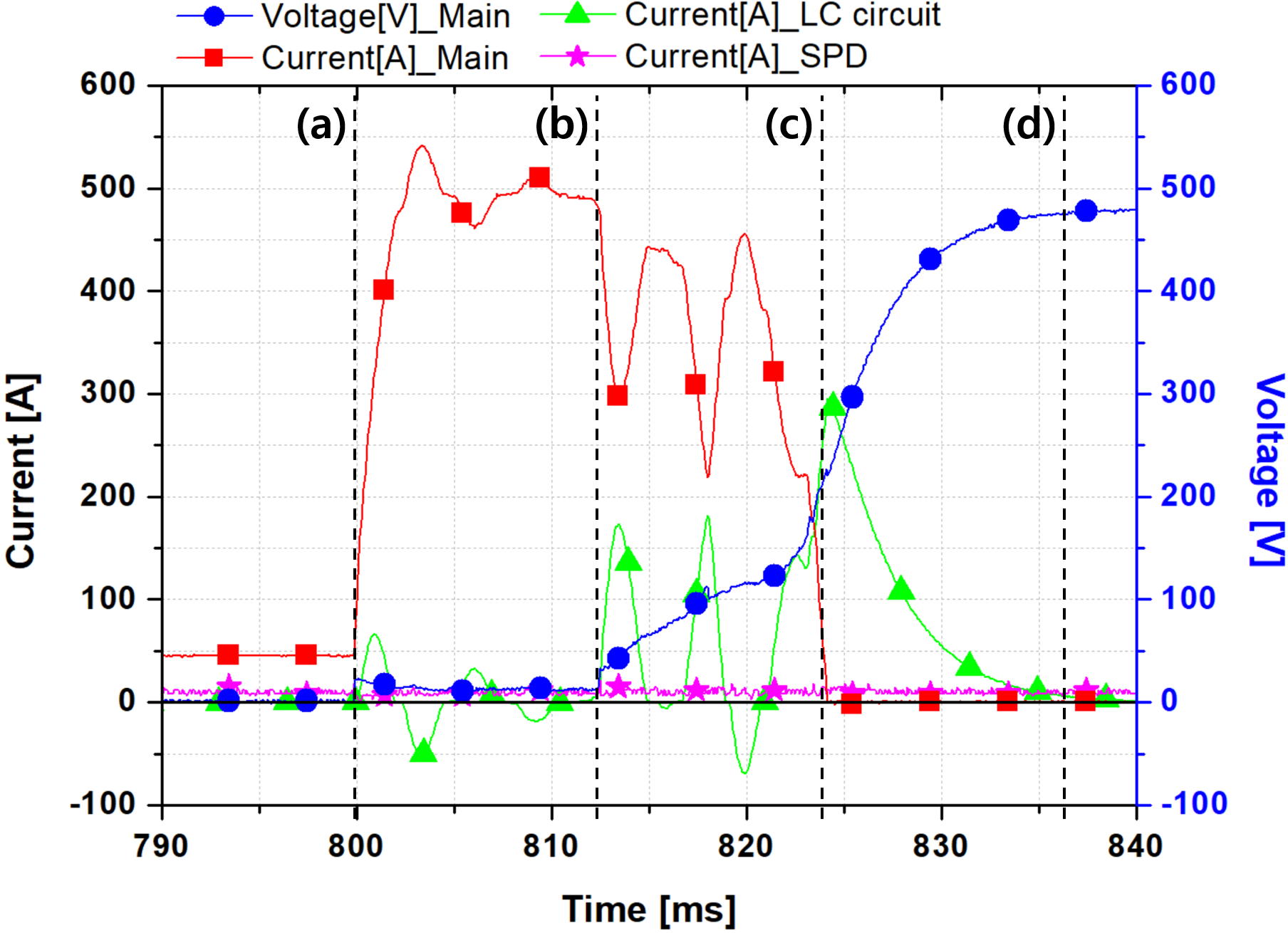### 2.3 Simulation modeling of DC circuit breaker using superconducting fault current limiter

Figure 3 is a circuit diagram of a superconducting DC breaker. We modeled the DC system with the same experi- mental conditions as the mechanical DC circuit breaker by simulation through the PSCAD/EMTDC program. The rated voltage and current are 500 V, 50 A. The configuration is designed with a solar power with DC power, L is the line impedance, and the Superconducting module is a superconducting element. We made a superconducting fault-current-limiter using the GdBCO material. We confirmed the parameters the operating characteristics input to the superconducting module of the simulation through the experiments. We obtained data on the rate of change from the superconducting state to the normal state and the data of the generated resistance through experiments. The data of the superconducting module were formulated and applied to the PSCAD/EMTDC program (6). Arc model is a model input by formulating arc characteristics that can be realized in simulation. In particular, the superconducting fault current limiter model was designed based on Equation (1) and the Arc model based on Equation (2). $R_{SC}$ is quenching resistance of the superconductor. $R_{m}$ is the maximum quenching resistance of the superconductor. $T_{SC}$ is the time constant for the operating characteristics of the fault current. ${g}_{m}$ is the arc conductance. $\tau_{m}$ is the arc time constant. $U_{}arc$ is the arc voltage. $I_{}arc$ is the arc current. $P_{o}$ is the cooling power constant.

##### (1)
$R_{SC}(t)=\left\{\dfrac{0(t<t_{quen\chi}ng)}{R_{m}\sqrt{[1-\exp(-\dfrac{t}{T_{SC}})^{1/2}(t_{quen\chi}ng<t)]}}\right\}$

##### (2)
$\dfrac{1}{{g}_{m}}\dfrac{d{g}_{m}}{dt}=\dfrac{1}{\tau_{m}}(\dfrac{v_{}arc\bullet i_{}arc}{P_{o}}-1)$

Mechanical switch is a mechanical DC circuit breaker, and an LC divergent oscillation circuit in which a reactor and a capacitor are connected in series are connected in parallel. In addition, SPD is connected in parallel to extinguish residual current. In the simulation, we designed the SPD with a smaller blocking capacity than SA. Normal load and fault load were configured. The simulation was compared and analyzed with two models.

그림 3 초전도 DC 차단기의 회로 구성

Fig. 3 Configuration circuit of superconducting DC circuit breaker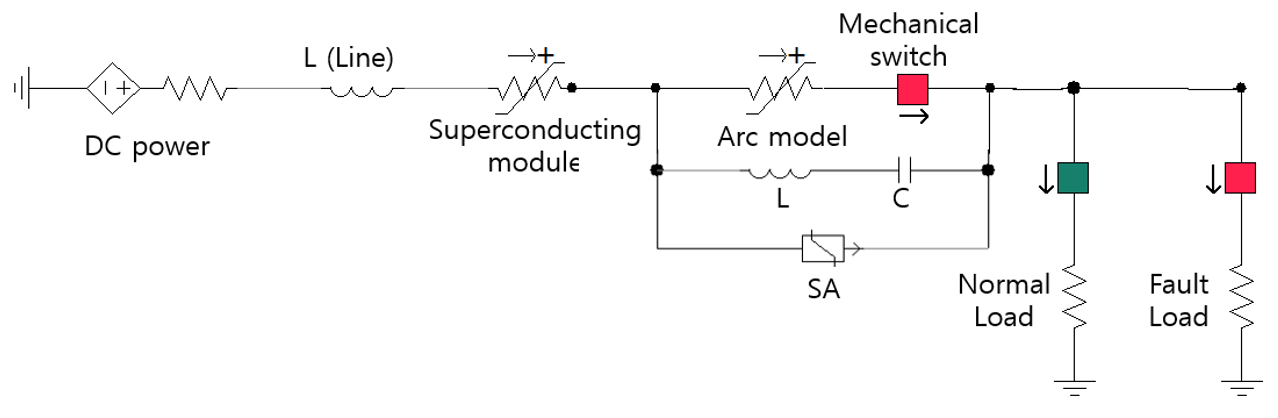### 2.4 Simulation results

그림 4 실험 결과를 바탕으로 설계된 시뮬레이션 모델의 동작 특성 그래프

Fig. 4 Graph of the operating characteristics of the simulation model designed based on the experimental results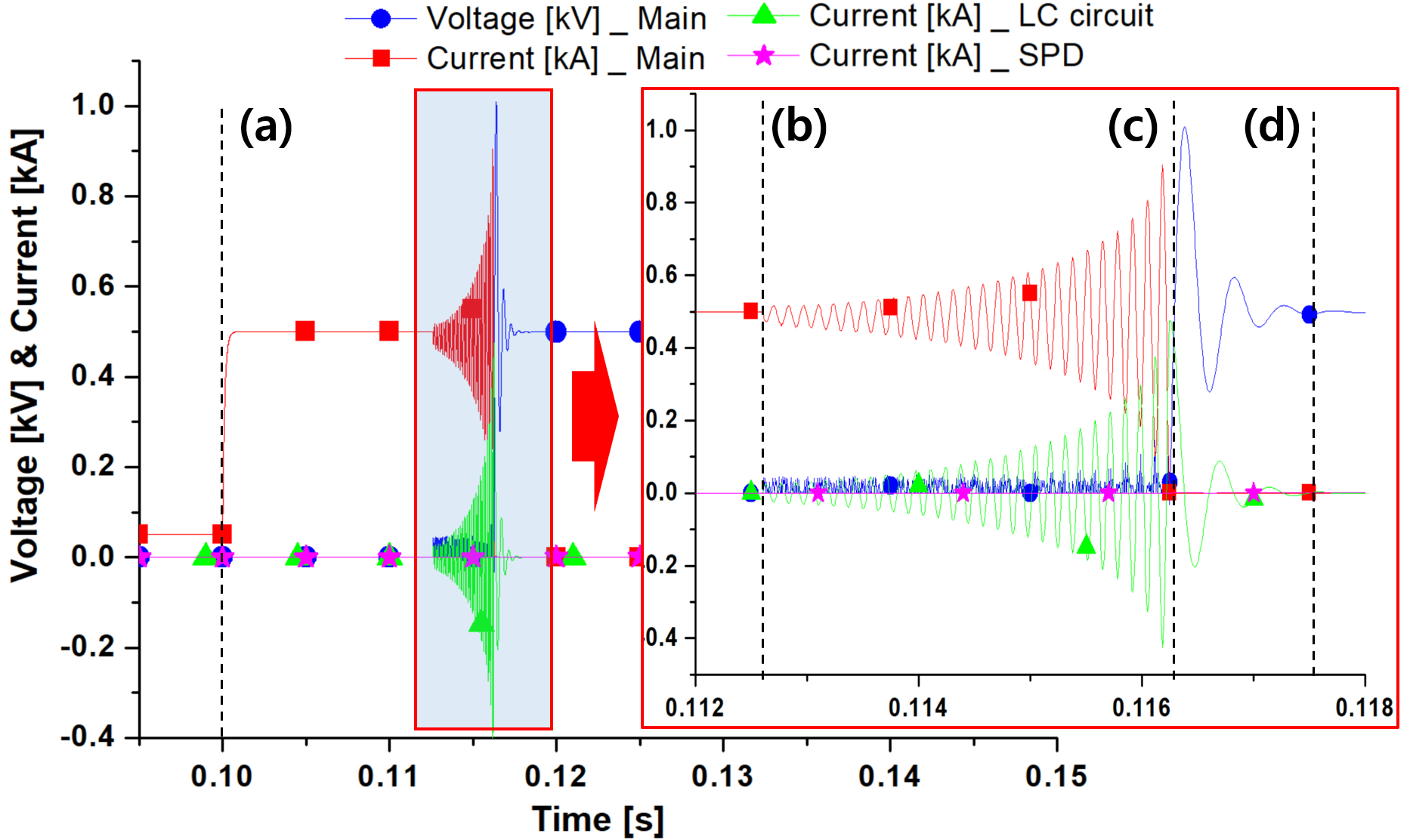Table 1 shows the results of experiments and simulations. ① is a model composed of only mechanical DC circuit breaker. ② is a model composed of the mechanical DC circuit breaker and LC divergent oscillation circuit. ③ is a model composed of the mechanical DC circuit breaker, LC divergent oscillation circuit and superconducting module. The Figure 1 is the experiment ①. The Figure 2 is the experiment ①+②. The Figure 3 is the simulation ①+②. The Figure 4 is the simulation ①+②+③. In each case model, the time when the artificial zero point occurred and the time when the fault current was completely cut-off were summarized.

그림 5 실험 결과를 바탕으로 설계된 두 번째 시뮬레이션 모델의 동작 특성 그래프

Fig. 5 Graph of the operating characteristics of the second simulation model designed based on the experimental results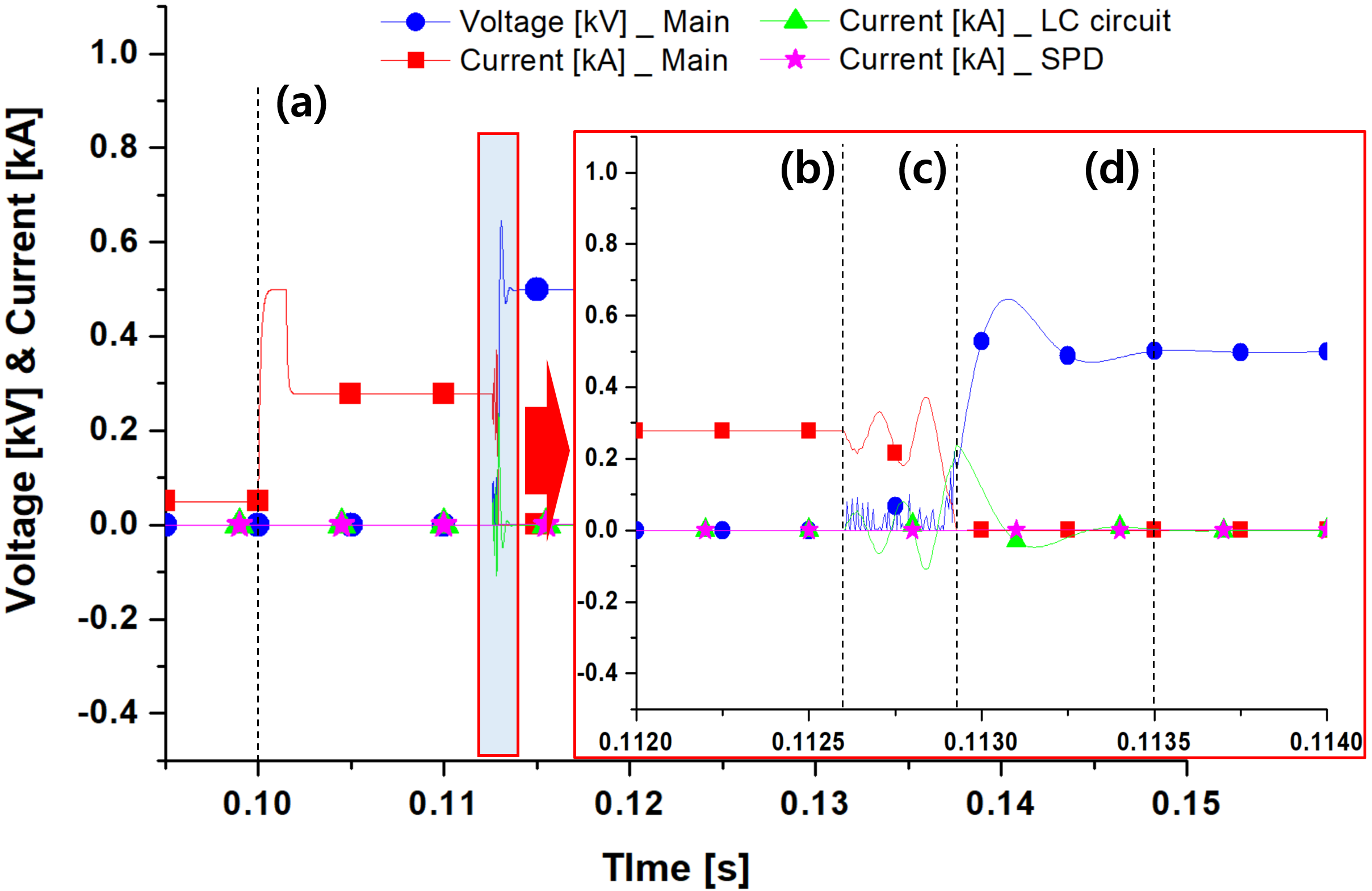그림 6 초전도 소자의 유무에 따른 기계식 DC 차단기의 전력 부담 비교 그래프

Fig. 6 Graph of the power burden of the mechanical DC circuit breaker Depending on the presence or absence of a superconducting element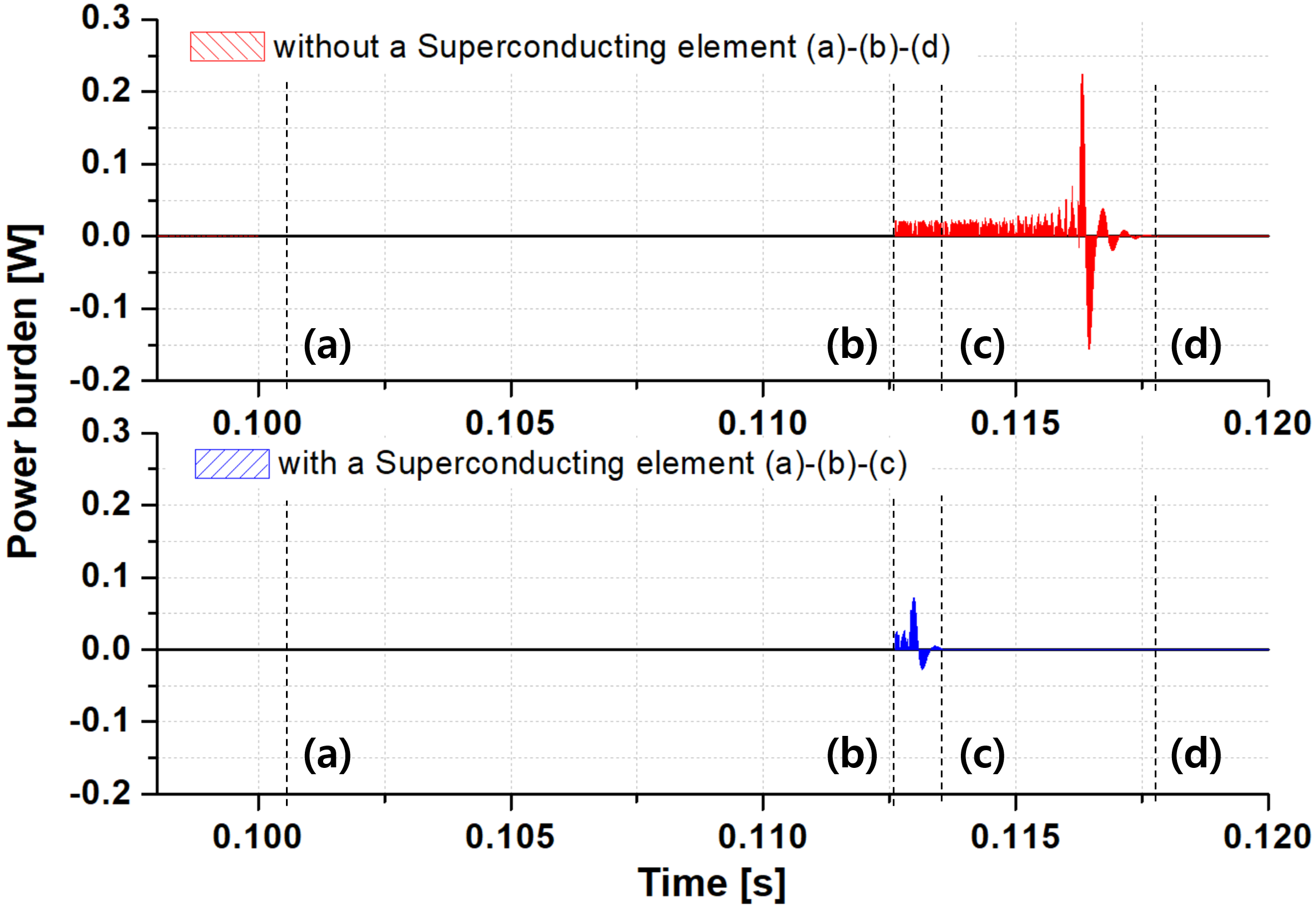### 2.5 Power burden

Figure 6 shows the comparison data of the power burden according to the application of superconducting elements in a graph based on the simulation data of Figure 4 and 5. Figure 6-(a) shows the point of the time when the fault occurred, and it was about 0.1 s. Figure 6-(b) is the point of the time when the circuit breaker is opened, and it is set identically to compare the data on the power burden. Its value was about 0.1126 s. The time of the mechanical contacts opening depended on the opening characteristic of the BK63H DC circuit breaker. Figure 6-(c) is the point of the time when the blocking when there is a superconducting element is completed. Its value was about 0.1135 s. The power burden at this time was about 0.068 W. Figure 6-(d) is the point of the time when blocking is completed when there is no superconducting element, and the value is about 0.1181 s. The power burden at this time was about 0.092 W. Depending on the presence or absence of a superconducting element, the following operating characteristics were positively generated. First, the growth rate of DC fault current was suppressed by about 55.8 %. Second, the level of the cut-off current from the DC circuit breaker's position was formed to be low. Third, the voltage level of the TRV(transient recovery voltage) was formed lower by 64.6 %. Fourth, it was confirmed that the power burden of the mechanical DC circuit breaker was reduced by about 73.9 %.

표 1 실험과 시뮬레이션 결과표

Table 1 Results of the experiment and simulation

 Composition Artificial current zero-point [ms] Cut-off operation completed [ms] Experiment ① - 16.1 ①+② 24.3 37.3 Simulation ①+② 16.2 17.5 ①+②+③ 12.9 13.5

## 3. 결 론

We analyzed the operation characteristics of superconducting DC circuit breaker by simulation. We confirmed the operation characteristics of the mechanical DC circuit breaker and the LC divergent oscillation circuit except for the superconducting module through experiments and the combined model of the superconducting module was confirmed through simulation. The simulation model of the mechanical DC circuit breaker was applied the operation characteristics of the general mechanical DC circuit breaker which is the actual equipment of the photovoltaic power generation. In addition, we manufactured the LC divergent oscillation circuit and applied to the mechanical DC circuit breaker. We confirmed the operation characteristics of the mechanical DC circuit breaker according to the presence or absence of the LC divergent oscillation circuit through the experiment. Based on the experimental data, a simulation was constructed and a superconducting module was applied. As a result, it was confirmed that the growth slope of the fault current decreased according to the quenching of the super- conducting module. In addition, the method of the zero point generation of the LC divergent oscillation circuit helped the quenching operation of the superconducting module to minimize the power burden of the mechanical DC circuit breaker. we were able to confirm the improvement of the superconducting module and we intend to conduct the experiment by making a superconducting model based on the simulation data.

### Acknowledgements

This research was supported by Korea Electric Power corporation [grant number: R21XO01-32]

### References

1
M. Rautkivi, M. Kruisdijk, 2013, Future Market Design for Reliable Electricity Systems in Europe, present. at the POWER GEN Europe’132
F. Zheng, C. Deng, L. Chen, S. Li, Y. Liu, Y. Liao, 2015, Transient Performance Improvement of Microgrid by a Resistive Superconducting Fault Current Limiter, IEEE Transactions on Applied Superconductivity, Vol. 253
B. Xiang Zhiyuan, L. Yingsan Geng, S. Yanabu, 2015, DC Circuit Breaker Using HTS for Current Limiting, IEEE transac- tions on applied superconductivity, Vol. 25, No. 2, pp. 1-74
B. Xiang, M. Junaid, L. Gao, Z. Liu, Y. Geng, J. Wang, S. Yanabu, 2019, Effects of short circuit currents on quench and recovery properties of YBCO Tapes for DC SFCL, IEEE transactions on Applied Superconductivity, Vol. 29, No. 2, pp. 56007065
P. H. Schavemaker, L. V. D. Sluis, 2000, An improved Mayr-type Arc model based on current-zero measurements, IEEE trans on power delivery, vol. 15, Vol. no. 26
S. H Hwang, 2017, A Study on Superconducting Interruption System for Reducing of Power Burden in DC Syste, Master’s Thesis Chosun University Graduate School## 저자소개

Cur- rently completing the PhD program at the same graduate school.

Regular member of the Korean Electrical Society Major Research Areas of Interest : Applications of superconducting Power system, Applications of DC circuit breaker

Tel: 062-230-7054

E-mail : sangyong4400@gmail.com

Currently Professor, Department of Electrical Engineering, Chosun University, 2021 ~ Vice President of the Korean Electrical Society.

Major Research Areas of Interest : Applications of superconducting Power system, Applications of DC circuit breaker, wireless power transmission.

Tel: 062-230-7025

E-mail : hyosang@chosun.ac.kr